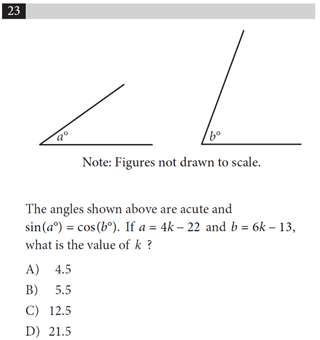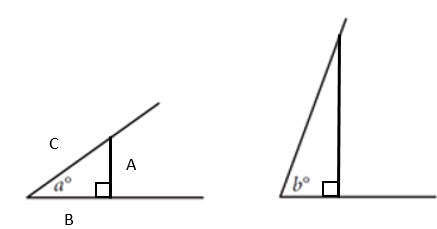Search
• nokwareknight

# Closer Look at an SAT Acute Complementary Angles Problem

Updated: Mar 9

Earlier this week, we took a look at the following problem. This comes from practice test 3, section 3 (no calculator), question #23.The written explanation to the solution provided by SAT starts as “Since the angles are acute and sin(a) = cos(b), it follows from the complementary angle property that sines and cosines that a + b = 90.

Taking this first step feels a bit like waving a magic wand. Yes, it moves us closer to the answer. And it might help to remember for the exam that if sin(a) = cos(b) in an acute triangle, we can assume that a and b are two different acute angles in the same right triangle.

But how did we even get here? And when we say “complementary angle property” of an acute triangle, isn’t that about two different angles in the same triangle (and not in two different triangles like in the given question)?

Let’s slow down to get a sense of why using the complementary angle property is a good first step in working towards an answer.

When we are using sin and cos of angles, we are referencing the sides of right triangles. We can create our right triangles by adding a couple of straight lines to the images provided in the question.Now that the sides are labeled, sin(a) can be more formally defined asThey also tell us that sin(a) = cos(b). SoSo let’s put sides A and C in the appropriate positions of the second triangle.Since, in an acute right triangle......this relationship will also hold for the first triangle, which also (proportionately) has sides A and C.Now we have angles a, b, and 90 degrees in the same triangle. So, at least proportionately, we are dealing with two of the same triangles (or similar triangles). Since all angles in a triangle add up to 180 degrees, we have

a + b + 90 = 180

Simplify by subtracting 90 from both sides.

a + b = 90

Now we can substitute in a and b.

a + b = 90

a = 4k - 22

b = 6k - 13

And so

(4k - 22) + (6k - 13) = 90

You can solve for k from there.

-Nokware Knight